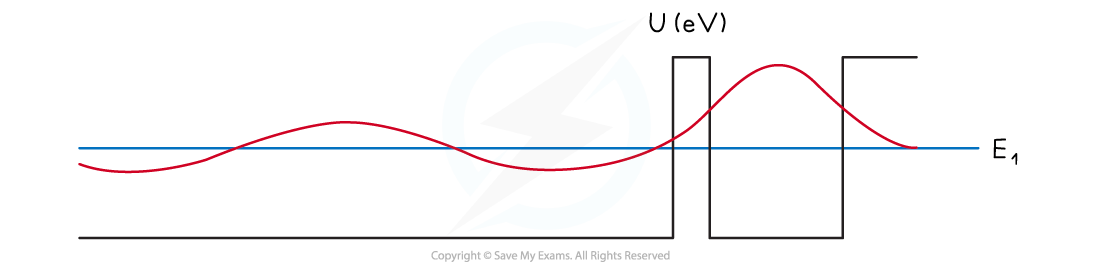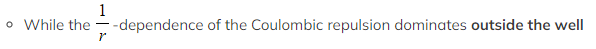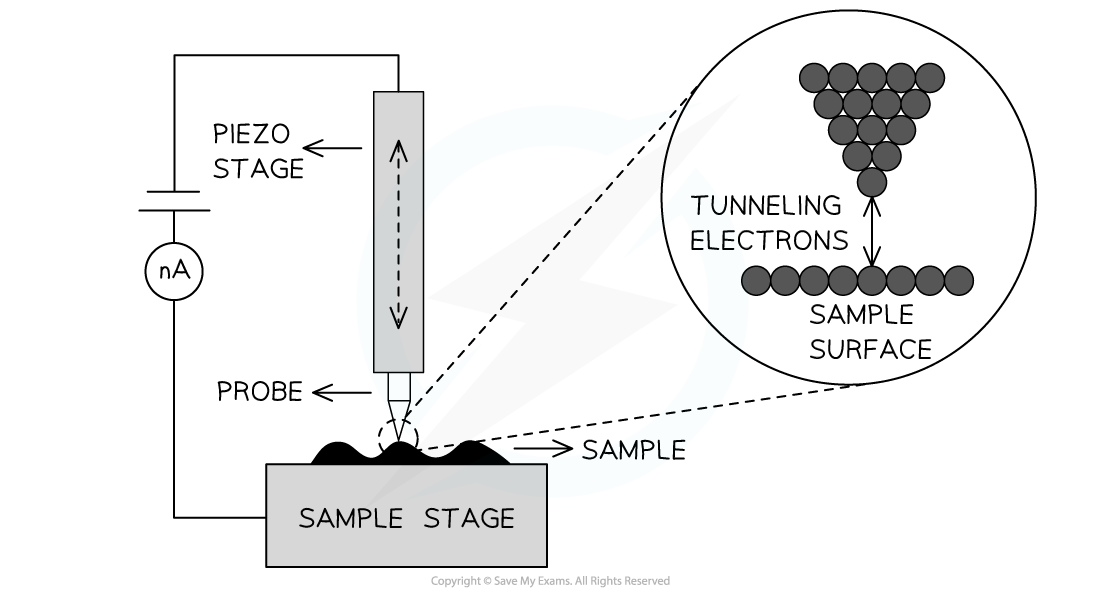# IB DP Physics: HL复习笔记12.1.10 Tunnelling

### Tunnelling

• Single potential wells lead to quantised energy levels and their associated wavefunctions
• The wavefunction extends throughout space
• However, for infinitely deep square wells the wavefunctions are localised within the well region
• The probability of finding the quantum particle at the barrier is zero
• For a finite barrier, the wavefunction can penetrate the barrier
• So, the particle has some probability of being in a “classically forbidden region”
• If there are two well-like regions, the solution of Schrodinger’s equation gives an energy level and wavefunction that extends over the whole region of the potential well
• When the red wave function is squared it gives the probability of finding the particle in a particular region of space
• Since the wave function extends through the barrier this means there is a finite probability of finding the particle in either of the two well regionsA thin barrier or classically forbidden region can result in tunnelling

• Consequently, if a quantum particle were placed in the narrow well on the right, it is possible at some time later to find it in the region on the left
• The particle is said to have tunnelled through the narrow barrier

#### Tunnelling & Alpha Decay

• The strong nuclear force within the nucleus is represented by the square well
•Nucleons in the nucleus have quantised energy levels and wave functions
• An alpha particle can gain energy and occupy an excited energy level where the barrier width is smaller
• As a result, the alpha particle can tunnel through the classically forbidden region
• This greatly increases the probability of the alpha particle being emittedAlpha decay through quantum mechanical tunnelling

#### Uses of Quantum Tunnelling

• Quantum tunnelling is utilised in several systems, for example in:
• Semiconductor devices
• Fusion reactions in the Sun
• A scanning tunnelling microscope
• In one mode of operation of a scanning tunnelling microscope, a sharp point, one atom thick, is maintained close to a surface
• This is so that a small tunnelling current between the tip and the surface remains constant
• In this case, the gap between the tip and the sample surface acts as the barrier that the electrons must tunnel through
• The tip is moved up and down and across the surface by piezoelectric transducers allowing the sample surface to be mapped outSimplified schematic of a scanning tunnelling microscope